Checkout JEE MAINS 2022 Question Paper Analysis : Checkout JEE MAINS 2022 Question Paper Analysis :

# Area Of A Kite

Rhombus- A rhombus is a quadrilateral in which all the four sides are of equal length. Unlike a square, none of the interior angles of a rhombus is not

$$\begin{array}{l}90^{\circ}\end{array}$$
in measure.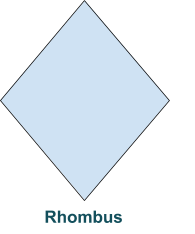## Kite

The diagonals of a kite are perpendicular.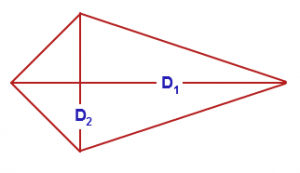## Area of a Kite

If we know the diagonals of a kite, it is possible to calculate the area of a kite.

Let

$$\begin{array}{l}D_{1}\end{array}$$
and
$$\begin{array}{l}D_{2}\end{array}$$
be the long and short diagonals of the kite, respectively.

Then the area of a kite is given by –

A =

$$\begin{array}{l}\frac{1}{2}D_{1}D_{2}\end{array}$$

## Proof for Area of a Kite

Let us consider a kite ABCD. Let diagonals AB(

$$\begin{array}{l}D_{1}\end{array}$$
) and CD(
$$\begin{array}{l}D_{2}) \end{array}$$
meet at point E. Thus we see that a diagonal divides a kite into two triangles.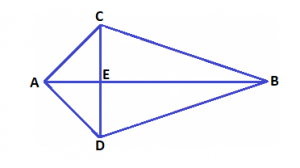In the figure given above, we see that Diagonal AB divides a kite in two triangle ACB and ADB.

Thus area of traingles equal to –

$$\begin{array}{l}Area (\bigtriangleup ACB) = \frac{1}{2} \times AB \times CE\end{array}$$

and,

$$\begin{array}{l}Area (\bigtriangleup ADB) = \frac{1}{2} \times AB \times DE\end{array}$$

Area of a Kite

$$\begin{array}{l} = Area (\bigtriangleup ACB) + Area (\bigtriangleup ADB)= \frac{1}{2} \times AB \times CE + \frac{1}{2} \times AB \times DE\end{array}$$

Area of a Kite

$$\begin{array}{l} = \frac{1}{2} \times AB \times (CE + DE)\end{array}$$

Area of a Kite

$$\begin{array}{l}= \frac{1}{2} \times AB \times (CD) = \frac{1}{2} \times D_{1} \times D_{2}\end{array}$$

 Solved Example- Example: Find the area of kite whose diagonals are 20 cm and 15 cm. Solution: We know, Area of a kite $$\begin{array}{l} = \frac{1}{2}D_{1}D_{2}\end{array}$$ Area $$\begin{array}{l} = \frac{1}{2} \times 20 \times 15 \;\;cm^{2}\end{array}$$ $$\begin{array}{l}= 150 cm^{2}\end{array}$$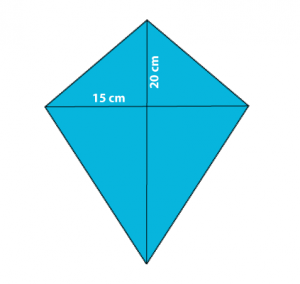If lengths of unequal sides are given, using Pythagoras theorem, the length of diagonals can be found.

 Example: The sides of a kite are given as follows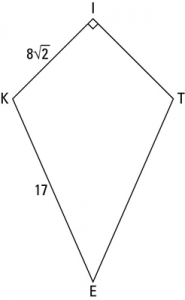Find the area of a kite. Solution: Given IK = $$\begin{array}{l}8\sqrt{2}\end{array}$$KE = 17 Construction- Draw in segment KT and segment IE as shown in the figure alongside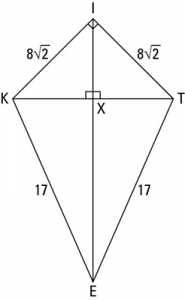To find the area of a kite, first, we need to calculate the length of the diagonals KT, EI. In Triangle IKX, it is clear that $$\begin{array}{l}\angle XKI = XIK\end{array}$$ So, the length KX = XI Using Pythagoras Theorem, we have $$\begin{array}{l}KX^{2}+XI^{2}= KI^{2}\end{array}$$ $$\begin{array}{l}XI^{2}+XI^{2}= KI^{2}\end{array}$$ $$\begin{array}{l}2 XI^{2}= \left ( 8\sqrt{2} \right )^{2}\end{array}$$ Therefore $$\begin{array}{l}XI = KX = 8\end{array}$$ units In Triangle KEX, using Pythagoras theorem we have $$\begin{array}{l}KX^{2}+XE^{2}= KE^{2}\end{array}$$ $$\begin{array}{l}8^{2} + XE^{2} = 17^{2}\end{array}$$ $$\begin{array}{l}XE^{2} = 289 – 64 = 225 \end{array}$$ Therefore $$\begin{array}{l}XE = 15\end{array}$$ units Thus the length of diagonals are – Diagonal KT = KX + XT = 8 + 8 = 16 units Diagonal IE = IX + XE = 8 + 15 = 23 units Area = $$\begin{array}{l}\frac{1}{2} \times D_{1} \times D_{2}\end{array}$$ $$\begin{array}{l}= \frac{1}{2} \times 16 \times 23 \;\; unit^{2}\end{array}$$ = $$\begin{array}{l}184 \; units^{2}\end{array}$$

This was all about kite. Learn various related concepts of topics like Quadrilateral, Trapezoid, Rhombus, Rectangle, Square, etc. in an engaging manner by visiting our site BYJU’S.